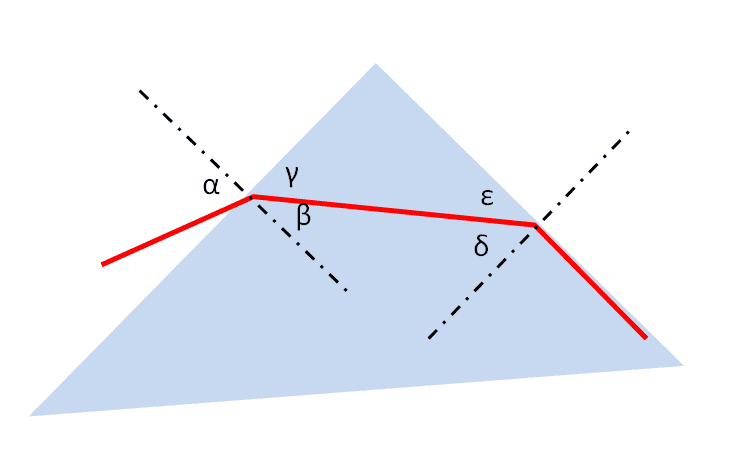# For which incidence angle the ray won't come out of the prism's surface...

## Homework Statement

A ray of light falls on the surface AC of a prism with a straight angle and with two sides equal. For which incidence angle the ray won't come out of the surface AB. The index of refraction for the prism is n=2.

sina/sinb=n2/n1

## The Attempt at a Solution

sinb=1 so sina=n2/n1=1/2 the angle is pi/6 rad. Am I right?

blue_leaf77
Homework Helper
Give us the related picture.

Give us the related picture.
http://s32.postimg.org/6fsfdz69x/image.png
I am adding: pi/6 rad is the angle of incidence. The first refracted angle is pi/3 rad. sin pi/3/sina=1/2
sin a=1.7. Where am I wrong?

Last edited:
blue_leaf77
Homework Helper
What is the prism apex angle? In the problem statement you said "straight angle", but what does straight mean in this case? Googling this term resulted in a straight angle being equal to 180 degrees.

What is the prism apex angle? In the problem statement you said "straight angle", but what does straight mean in this case? Googling this term resulted in a straight angle being equal to 180 degrees.
It is pi/2 rad or 90 degrees.

blue_leaf77
Homework Helper
Take a look at the picture below,since you know that exit angle at the last surface is 90 degrees, you should be able to calculate ##\delta##, then ##\epsilon##, afterwards ##\gamma## follows naturally from that upper triangle and so on till you find ##\alpha##.

sin sigma/sin 90=1/2=> sin sigma= pi/6 rad. beta=180-90-30=60=pi/3 rad. (as the angle of two extensions is pi/2.
sin alpha/ sin 60=2/1=> sin alpha/sqrt(3)/2=2=> sin aplha=sqrt (3)=1.7. I don't know where I am wrong.

Last edited:
blue_leaf77
Homework Helper

Just did it.

blue_leaf77
Homework Helper
This potentially means that any incident angle on a right angle prism with ##n=2## will result in a total internal reflection at the second surface and hence no light can be transmitted.

Last edited:
This potentially means that any incident angle on a right angle prism will result in a total internal reflection at the second surface and hence no light can be transmitted.
What about the two equal sides of the prism. i didn't use this fact anywhere.

blue_leaf77
\begin{aligned} \sin \alpha &= n \sin\beta = n \sin(90^o-\delta) \\ &= n\cos \delta = n \sqrt{1-\sin^2 \delta} \end{aligned}
$$\sin \alpha = n \sqrt{1-\frac{\sin^2 \eta}{n^2}} \\ \sin \alpha = \sqrt{n^2 - \sin^2 \eta}$$
•